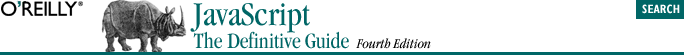home | O'Reilly's CD bookshelfs | FreeBSD | Linux | Cisco | Cisco Exam## 1.10. Exploring JavaScript

The way to really learn a new programming language is to write programs with it. As you read through this book, I encourage you to try out JavaScript features as you learn about them. There are a number of techniques that make it easy to experiment with JavaScript.

```<script>
document.write("<h2>Table of Fibonacci Numbers</h2>");
for (i=0, j=1, k=0, fib =0; i<50; i++, fib=j+k, j=k, k=fib){
document.write("Fibonacci ("  + i +  ") = " + fib);
document.write("<br>");
}
</script>```
`alert("Fibonacci ("  + i +  ") = " + fib); `

Note also that for simple JavaScript experiments like this, you can usually omit the <html>, <head>, and <body> tags in your HTML file.

```javascript:5%2
javascript:x = 3; (x < 5)? "x is less": "x is greater"
javascript:d = new Date( ); typeof d;